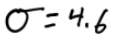# The Empirical Rule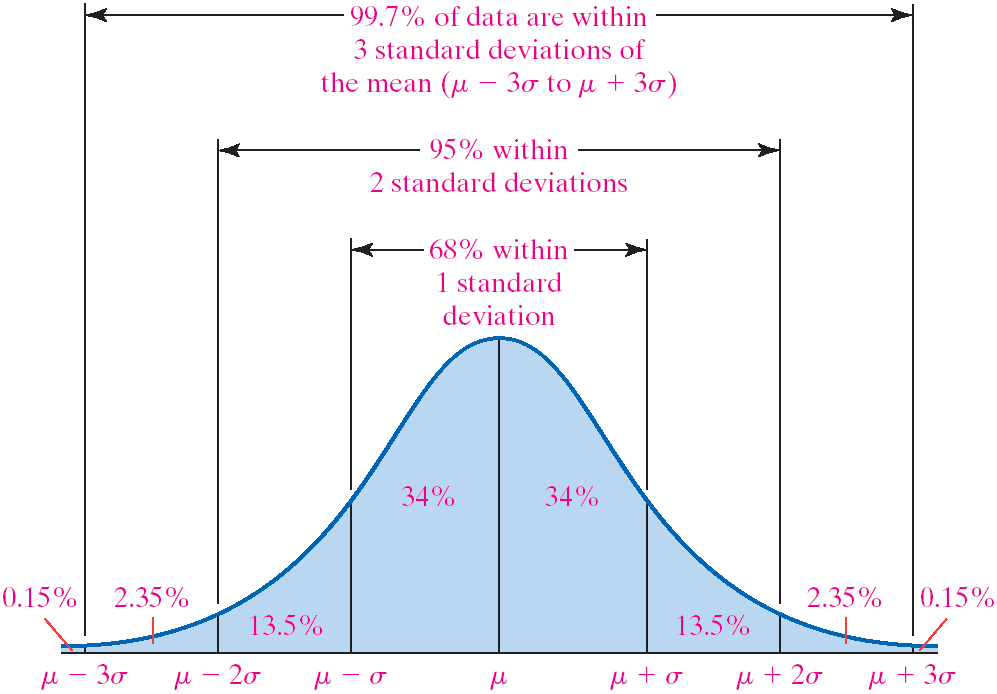# Z Scores (Standardized Score)

• How many standard deviations away from the mean your value x is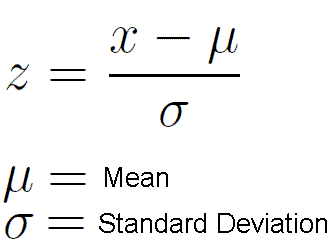# Using the Normal Table# Practice Questions

1. A study of college freshmen's study habits found that the time (in hours) that college freshmen use to study each week follows a normal distribution with a mean of 7.2 hours and a standard deviation of 5.3 hours
• How many hours do the students who study in the top 15% spend studying?

• The middle 68%?

• Top 15%: 12.5 hours

• Middle 68%: 1.9 hours to 12.5 hours1. Suppose that the weight of navel oranges is normally distributed with mean of 8 ounces, and standard deviation of 1.3 ounces. And the weights of Valencia oranges is normally distributed with mean of 9 ounces, and standard deviation of 1.6 ounces
• You grow a navel orange that weighs 9.5 ounces and a Valencia orange that weight 10.5 ounces, which should you enter in the giant fruit contest?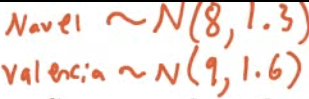• Z score for navel orange = (9.5-8)/1.3 = 1.1538

• Z score for Valencia orange = (10.5-9)/1.6 = 0.9375

• The weights of newborn children in the United States vary according to the Normal Distribution with mean 7.5 pounds and standard deviation 1.25 pounds.

• What is the probability that a baby chosen at random weighs less than 5.5 pounds at birth?

1. Draw a sketch1. Calculate Z score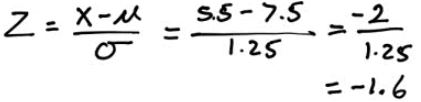2. Look up probability on the normal table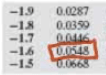3. The composite score of students on the ACT college entrance examination in a recent year had a Normal distribution with mean of 20.4 and standard deviation of 5.8

• What is the probability that a randomly chosen students scored 24 or higher on the ACT?

1. Sketch1. 2ND + VARS (DISTR) ➡️ 2: normalcdf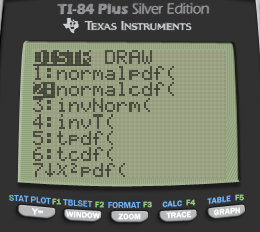2. Normalcdf(lower, upper, mean, standard deviation)• What is the probability that a randomly chosen student scored between a 19 and a 24 on the ACT?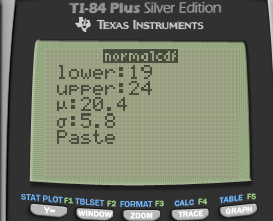• What score would someone in the 15th percentile have scored?

• Sketch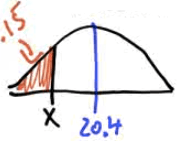3. Find the z value on the normal tableZ≈-1.035

4. Solve for x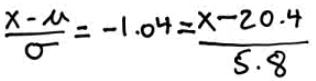5. Calculator: invNorm(area, mean, standard deviation)![ф TEns [NSTRUMENTS 14 „ гоямдтп си: ТАТЕ ](./media/image157.png)

6. Suppose that the mean height of men is 70 inches with a standard deviation of 3 inches. And suppose that the mean height for women is 65 inches with a standard deviation of 2.5 inches

• If the heights of men and women are Normally distributed, find the probability that a randomly selected woman is taller than a randomly selected man.

1. Sketch1. Find the necessary information
Mean SD
Men 70 3
Women 65 2.5
W-M 65-70 = -5 Sqrt(3^2+2.5^2) = 3.9
1. Calculate Z score2. Find the probability on the table and subtract that from 1

1-0.9015 = 0.0985 = 9.85%

• Suppose that the height (X) in inches, of adult men is a normal random variable with mean of 70 inches. If P (X>79) = 0.025

• What is the standard deviation of this random normal variable?

• Sketch3. Find the z score on the calculator: invNorm(area)

![ТИ-84 PIus S'tver Editj(n ф TEns [NSTRUMENTS гоямдта ](./media/image161.png)

4. Solve for SD